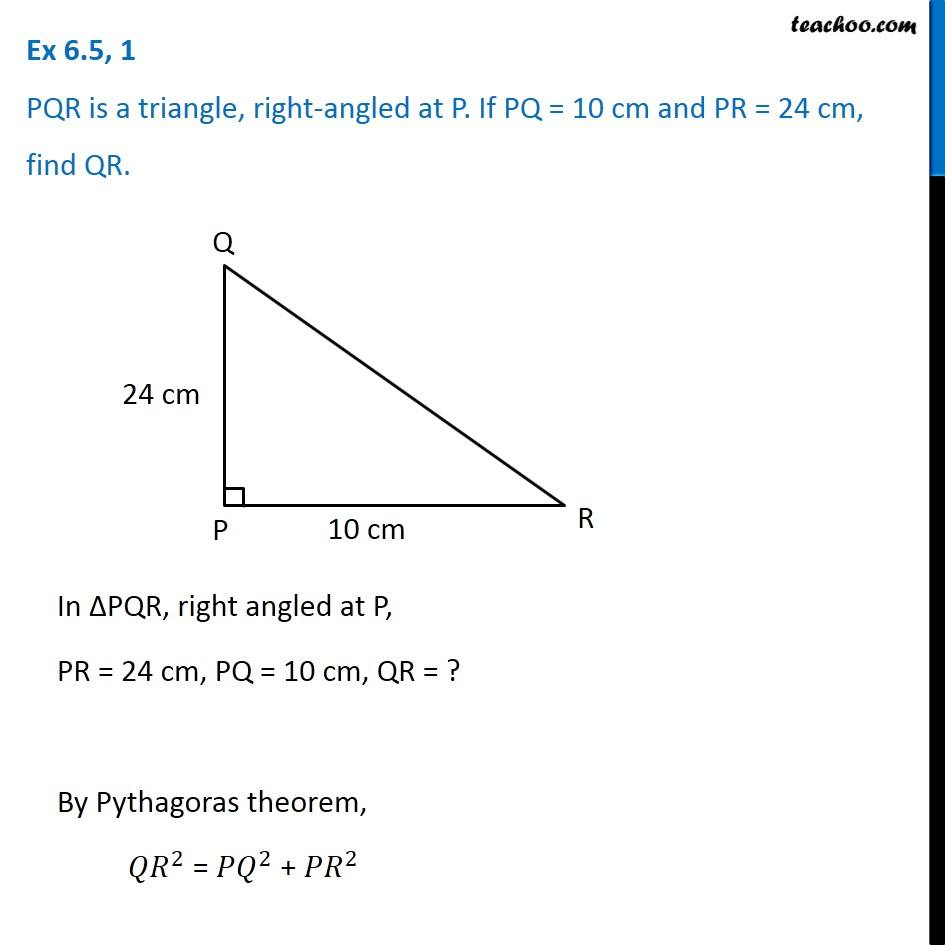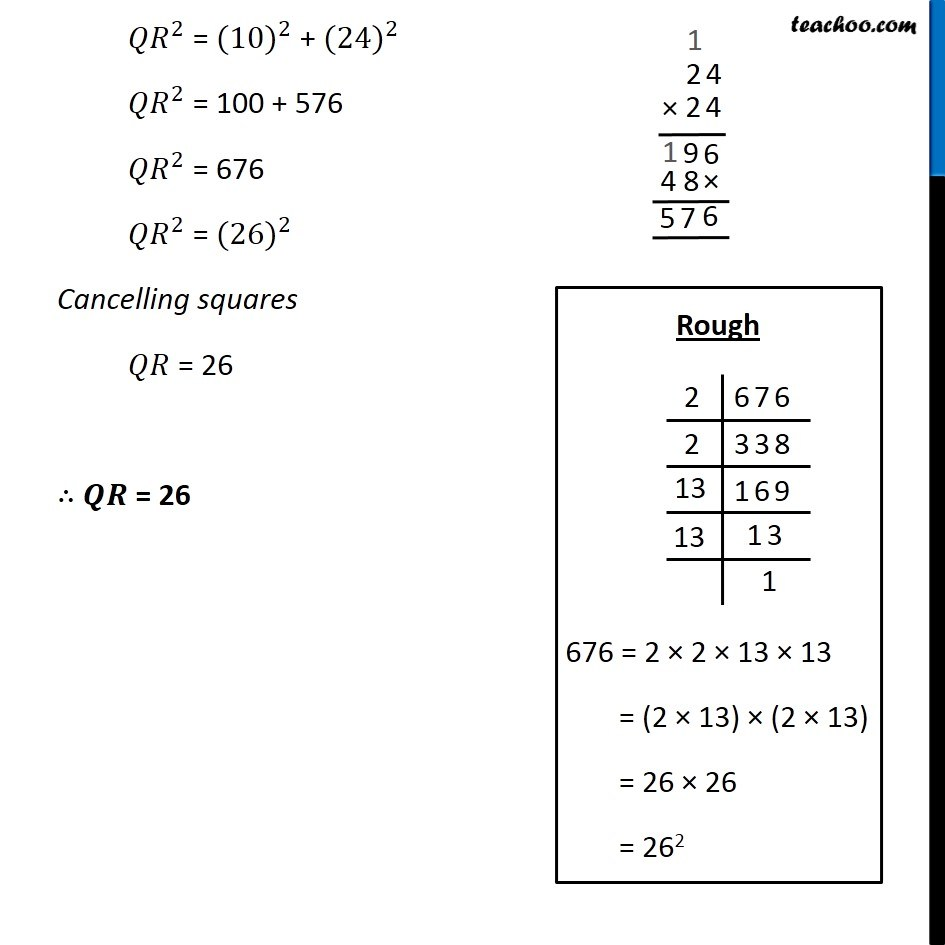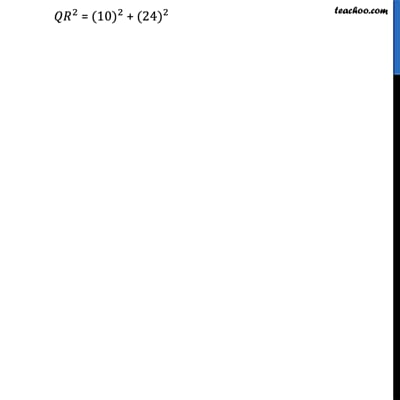Ex 6.5

Chapter 6 Class 7 Triangle and its Properties
Serial order wiseThis video is only available for Teachoo black users

Learn in your speed, with individual attention - Teachoo Maths 1-on-1 Class

### Transcript

Ex 6.5, 1 PQR is a triangle, right-angled at P. If PQ = 10 cm and PR = 24 cm, find QR.In ∆PQR, right angled at P, PR = 24 cm, PQ = 10 cm, QR = ? By Pythagoras theorem, 〖𝑄𝑅〗^2 = 〖𝑃𝑄〗^2 + 〖𝑃𝑅〗^2 〖𝑄𝑅〗^2 = 〖(10)〗^2 + 〖(24)〗^2 〖𝑄𝑅〗^2 = 100 + 576 〖𝑄𝑅〗^2 = 676 〖𝑄𝑅〗^2 = 〖(26)〗^2 Cancelling squares 𝑄𝑅 = 26 ∴ 𝑸𝑹 = 26 Rough 676 = 2 × 2 × 13 × 13 = (2 × 13) × (2 × 13) = 26 × 26 = 262